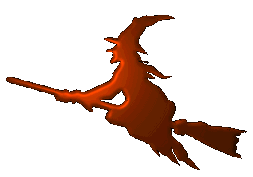Hex scattering database
Hex-dwba
This program computes the T-matrix from the first second order of the distorted wave Born approximation. The distorted wave Born approximation of the first order (DWBA-1) is similar to the well-known plane wave Born approximation. However, to speed up the convergence of the Born series (of which we are using just a single term in the first-order computation), part of the potential is excluded from the perurbative description and solved precisely. The spliting is the following $V = W + U \,$ where $$V$$ is the full potential felt by the projectile, $$U$$ is the so called "distorting potential" that will be solved precisely as mentioned above and explained below. This splitting has two consequences:
First of all, the free states are no longer free, so we cannot use the plane waves. Instead, one receives a distorted waves as a solution of the modified equation for partial waves $\left( -\frac{1}{2}\frac{\mathrm{d}^2}{\mathrm{d}r^2} +\frac{\ell(\ell+1)}{2r^2} +U(r) \right)\chi_{k\ell}(r) = E_{k}\chi_{k\ell}(r) \ .$
Secondly, the T-matrix receives two contributions now -- one from the scattering term and one as a correction due to the redefinition of the potentials. The result is the two-potential formula $T = (N+1) \left<\chi_f^{(-)}\psi_f\right|V-U_f\left|\mathcal{A}\Psi_i^{(+)}\right> + \left<\chi_f^{(-)}\psi_f\right|U_f\left|\psi_i\beta_i\right> \ .$
For details see the Doxygen documentation.
External links
Jakub Benda © 2017